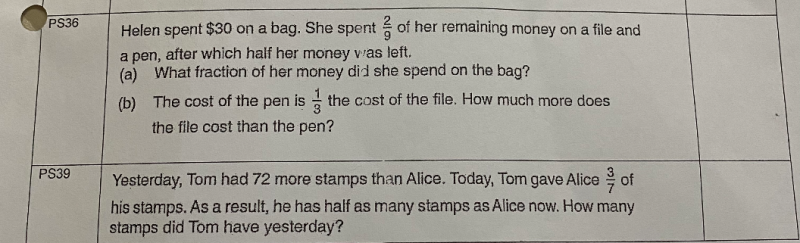# QuestionPS36(a) Let total money at first be u

<——————————–u————————————->

[–30—][——(2/9)(u-30)—][————-(1/2)u————— ]

< Bag ><— file + pen —-><———Final left —————>

< —— (7/9)(u-30)————–>

30 +  (2/9)(u-30) = (1/2) u    or alternatively,

(7/9)(u-30) = (1/2)u

(7/9)u – (210/9) = (1/2)u

(7/9)u – (1/2)u = 210/9

(14u – 9u)/18 = 70/3

u = 70/3   x   18/5   =  84

Hence fraction of money spent on bag = 30/84 = 5/14

(b)  Cost of file and pen = (2/9)(84-30) = 12

Let p be the cost of the file, then cost of pen = 1/3p

p + 1/3p = 12

(4/3)p = 12    =>   p = 12  x  (3/4) = 9

File = 9, Pen = 1/3(9) = 3, Difference = 9-3 = 6

Hence File is \$6 more than the Pen.

0 Replies 2 Likes ✔Accepted Answer

(a)

Assuming Helen has 9U money after spending \$30 on a bag.

30 + 9U = total money

Given

30 + 2U = ½( total money) = ½ (30 + 9U) multiply both side by 2 gives

60 + 4U = 30 + 9U

5U = 30

U = 6

30/ (30+9*6) =30/84 = 5/14

So 5/14 is the fraction of the money Helen spent on the bag

(b)

Cost of the pen = 1/3 of cost of file

Or you can say if pen costs 1P, then the file cost 3P, the difference is 2P

1P + 3P = 4P = 2 * 6=12

P = 3

2P= 6

So the file costs \$6 more than the pen.

====================================================================

If Tom has 7U stamp yesterday, then she left with  4U stamps today after giving 3U to Alice.

And Alice has 8U of stamps today. She has 5U yesterday.

7U = 5U + 72

2U = 72

U = 36

Tom has 7 *36  = 252 stamps yesterday.

Checking

Alice has 252-72 = 180 stamps yesterday

252 * 3/7 =108

Tom has 252-108 = 144 stamps today

Alice has 180 + 108 = 288 stamps today twice of what Tom has today.

0 Replies 1 Like

PS39  Alternative Solution:

Yesterday           Today left                New ratio

Tom                            u+72                 (4/7)(u+72)                 (1/2) p

u+72                 (8/7)(u+72)                    p

Alice                             u                     u + (3/7(u+72)                 p

(8/7)(u+72)u + (3/7(u+72)         ( x 7 for both sides)

8u + 576 = 7u + 3u +216

2u = 360

u = 180

Hence Tom has (180+72) = \$252  yesterday.

0 Replies 1 Like